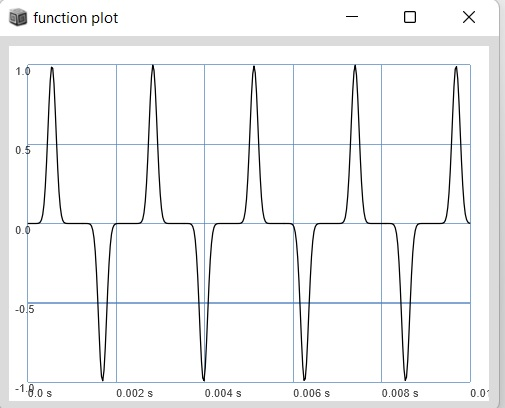# Pinch distortion

hey,
i was messing around with some distortion techniques from this thread Looking for starting point to write (or reuse) some "natural" sounding sound distortion - #2 by nathan
and tried out the pinch distortion as described by @nathan and replaced the odd number by a UGen.
i know that multiplying a signal by a modulator results in AM or Ring Modulation depending on the modulator (unipolar or bipolar). but does somebody know what happens theoretically when taking a signal to the power of something? thanks``````(
{
var sig = SinOsc.ar(440);
sig = (sig ** (LFNoise2.ar(2).range(1, 27)).lag(0.01)).fold2;
}.plot;
)
``````1 Like

Check out the waveshaping section (5.3) of Miller Puckette’s book. He explains the quadratic transfer function pretty well. What is really bizarre with SC is that a (UGen with a negative value)**2 will yield a negative value (see the Operators help file). This is really nice for wave shaping, but it took me looking at the help file to figure it out (it should always be positive when squared). So basically, you are designing a wave shaper that changes power randomly over time. It should sound pretty good, and the feature of the negative UGen yielding a negative value makes it work linearly as the power flips between positive and negative.

1 Like

hey, thanks for mentioning the book. I will have a deeper look at the waveshaping chapter.
so raising the signal to an odd power comes from the idea of wanting to have a negativ output value for a negative input value of the signal? which is not necessary in SC because of how the Ugens work ? so even numbers will do it as well?

EDIT: i think chapter 5.5.5. “waveshaping using an exponential function” is exactly with what we are dealing here.